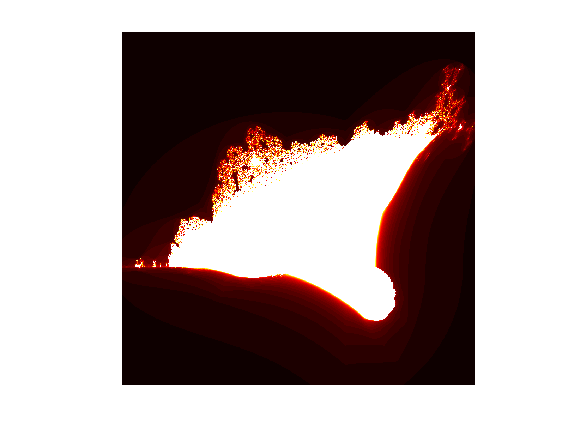• /
• # Burning ship fractal

on 6 Oct 2021
• 5
• 104
• 2
• 0
• 278
p = 1000;
x0 = -0.4;
y0 = -0.5;
r = 1.5;
k = 50;
x = linspace(x0-r, x0+r, p);
y = linspace(y0-r, y0+r, p);
[X,Y] = meshgrid(x, y);
Z = X + 1i*Y;
m = k*ones(size(Z));
z = Z;
for n=0:k
z = (abs(real(z)) + 1i*abs(imag(z))).^2+Z;
h = abs(z)>2;
z(h) = NaN;
m(h) = n;
end
imagesc(m)
colormap(hot(k))
axis equal
axis off